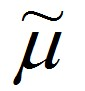# L-estimators

Statistical Analysis Techniques, Robust Estimators, Alternatives to OLSThe three main classes of robust estimators are M, L and R.  Robust estimators are resistant to outliers and when used in regression modelling, are robust to departures from the normality assumption.

L-estimators involve a linear combination of order statistics.  With univariate data, the sample median and α-trimmed mean are examples of L-estimators.  In regression, the focus is on the order of residuals.

### Least Trimmed of Squares (LTS)

The LTS method takes the following steps:
1.      Fit an OLS model to the data
2.      Store the residuals as its own variable
3.      Sort data set by the size of residuals
4.      Delete the observations that have the α smallest and α largest residuals, where 0<α<0.5
5.      Fit an OLS model to the remaining observations.

The LTS has a breakdown point equal to α.  The breakdown point is the percentage of the data that can be outlying before the fitted line is attracted to the outlying points.

### Least Median Squares (LMS)

This method involves finding the beta coefficients that minimise the median squared residual.  Since the objective function isn’t differentiable, the problem requires an algorithm to solve it.

Two types of algorithms have been suggested.  One of them is an expensive algorithm of order O(n2) (for one regressor) that searches all possible parameter values within plausible ranges to find the minimum median squared residual.  95% confidence intervals could be used as a criterion for coming up with bounds for the ranges.  The other type of algorithm has an order of O(nlog(n)) (for one regressor) and involves random sampling.

A problem with LMS other than its high computational cost is that it can be inaccurate because not all good points are used in the estimation.